## y ∝ √ x If y = 45 when x = 81 find, x when

Question

y

x
If
y
=
45
when
x
=
81
find,
x
when
y
=
100

in progress 0
3 months 2021-07-23T17:25:57+00:00 1 Answers 2 views 0

x = 400 when y = 100

Step-by-step explanation:

This is a question in relation to direct variation.

y ∝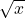y = kGiven that, y = 45, x = 81. Then;

45 = k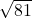45 = 9k

k =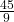k = 5

Thus the relationship among the variables is;

y = 5If y = 100, then;

100 = 5=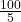= 20

x =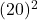x = 400

Therefore, x = 400 when y = 100.